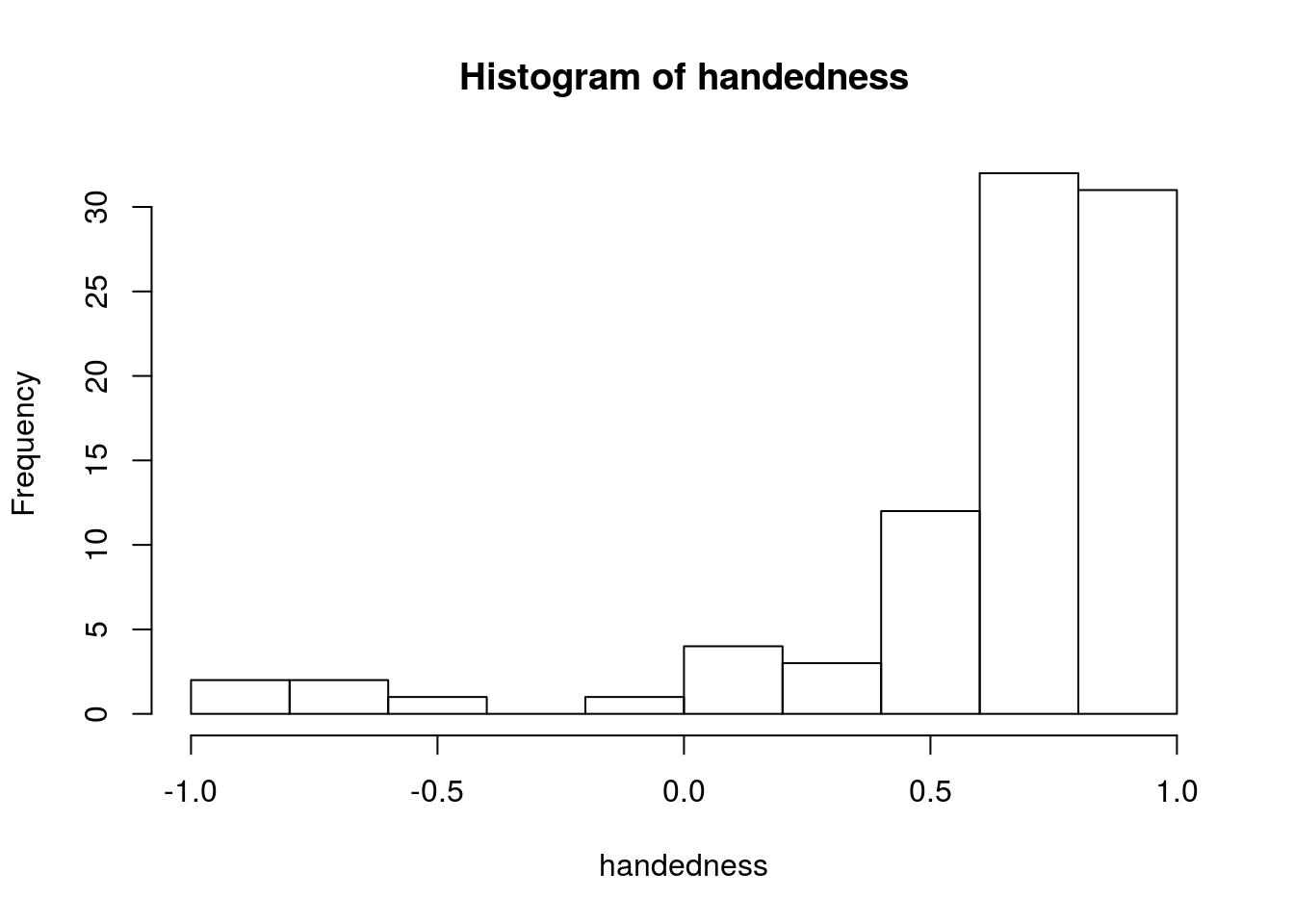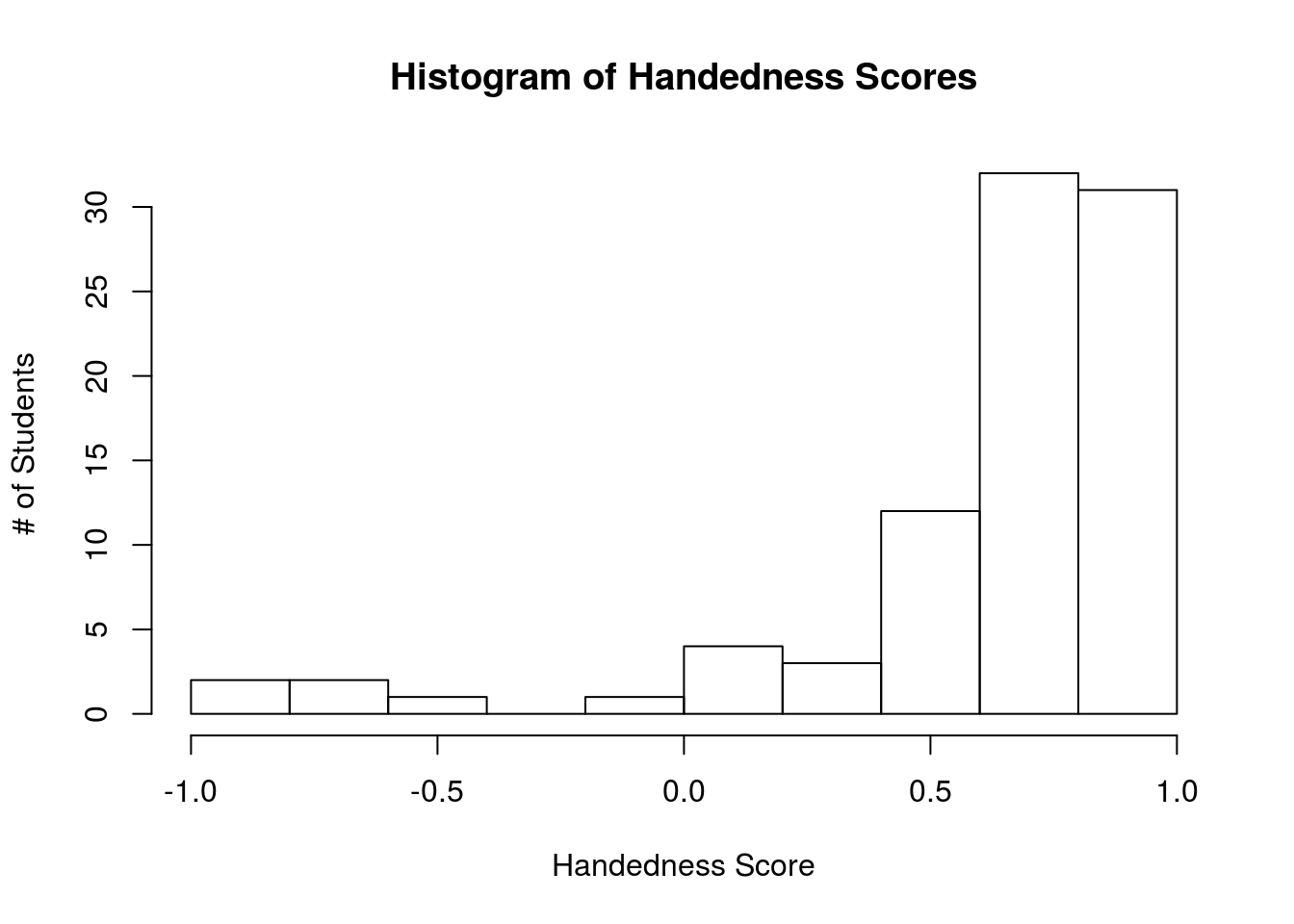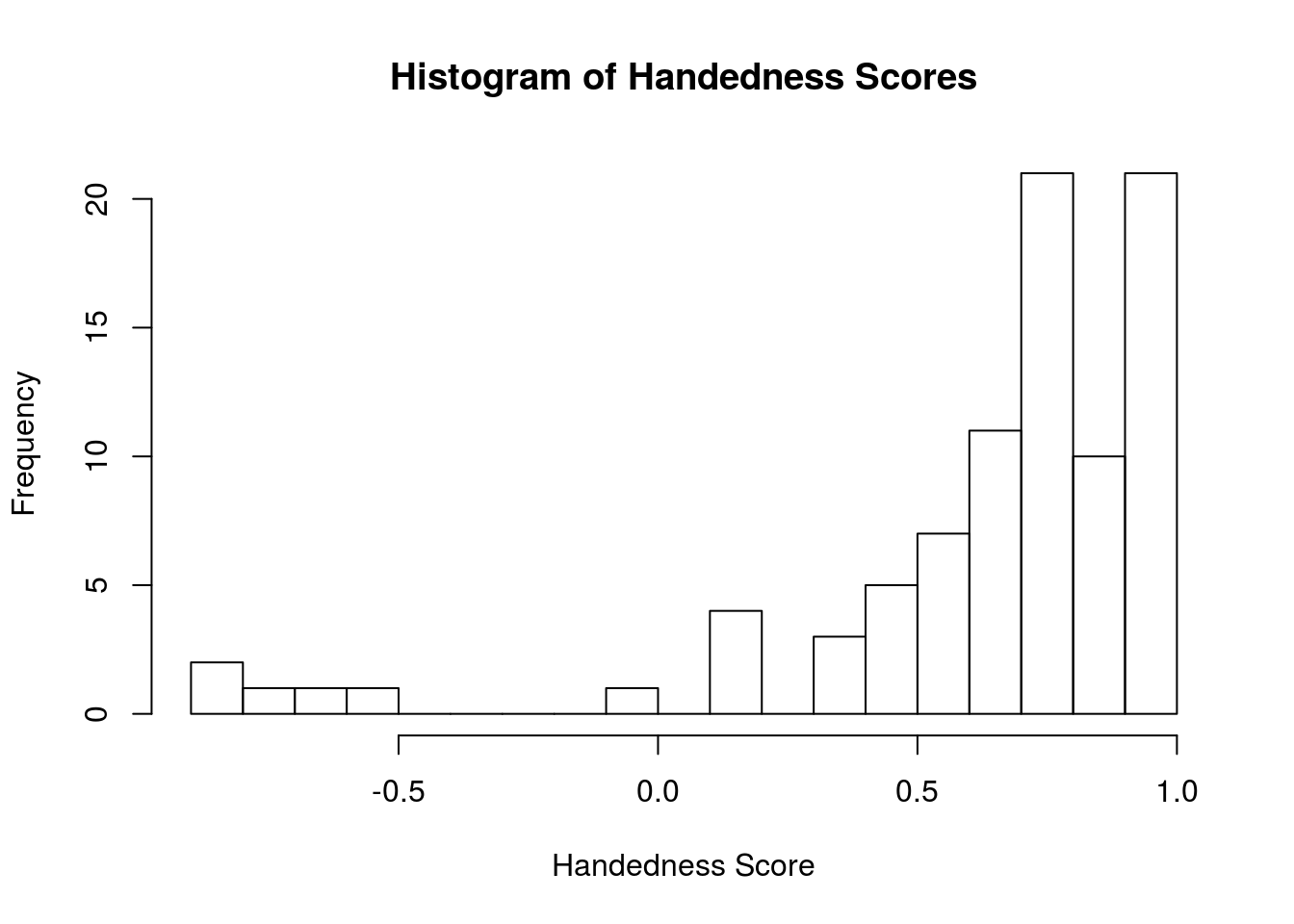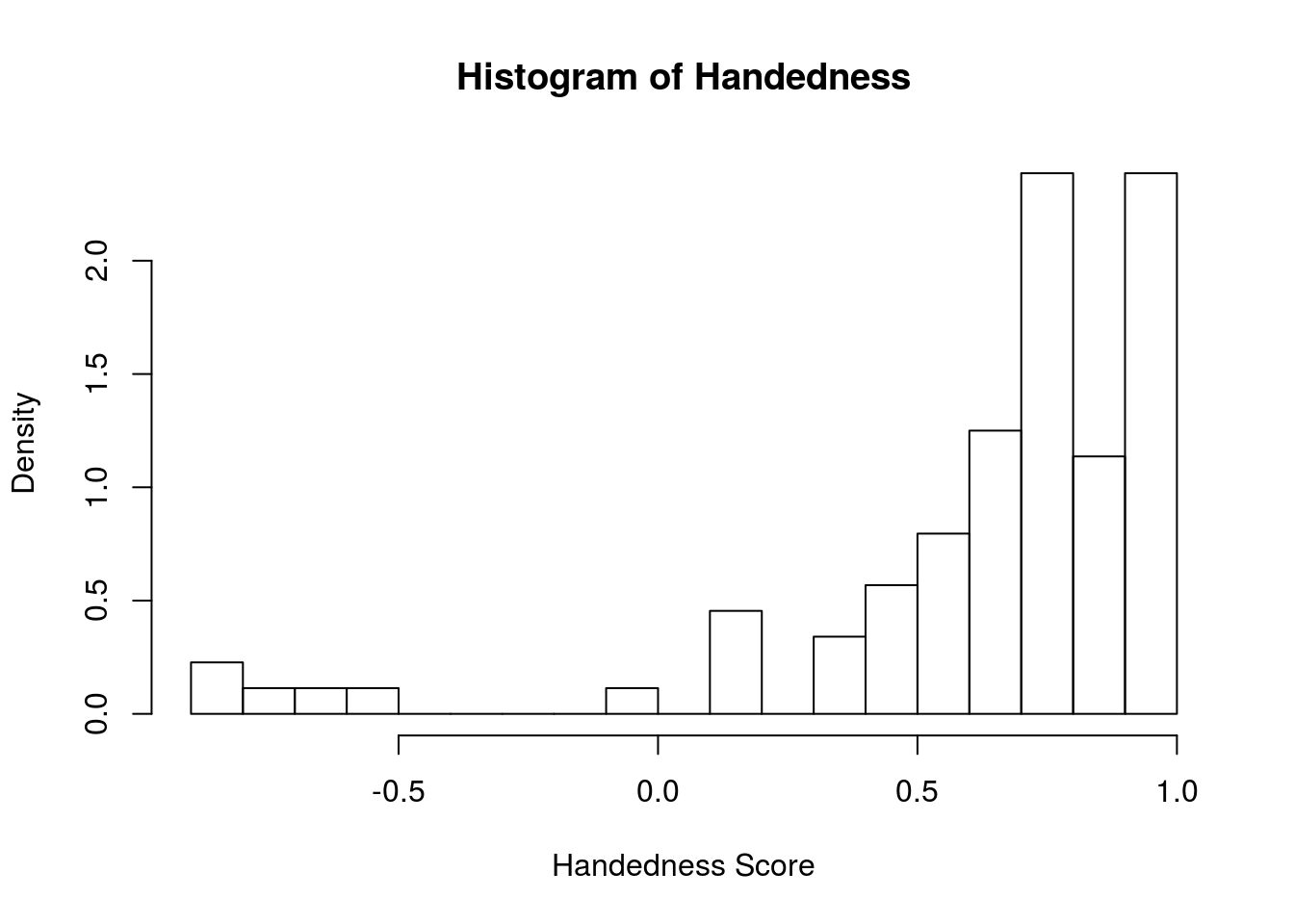This tutorial has three parts. In the first part, you’ll learn how to implement the summary statistics and graphics from class using R. In the second part, you’ll use what you’ve learned to explore a real-world dataset: the passenger manifest from the Titanic.

# Summary Stats and Graphics

In this section we’ll work with data from the student survey. Since results for this semester haven’t been collected yet, we’ll start with data from a previous semester:

``````#You should have data.table installed from the first R tutorial.
#  if not, run the following line of code before library:
#  install.packages("data.table")
library(data.table)

Adding and subtracting columns from a `data.table` is done with the special assignment operator `:=`, which I read as “defined as”. There are other ways to assign and remove columns from a `data.table` or `data.frame`, but there are issues with this approach; if you’re interested, see here, here and here for more background on this issue.

Let’s add a column to `survey` that is the ratio of `height` to `handspan`:

``survey[ , height_handspan_ratio := height/handspan]``

As always with `data.table`s, when we’re doing an operation specifying something about columns, we do so in the second argument (often referred to as simply `j`) within the square brackets `[]`. The first argument (similarly, `i`) tells R which rows we’re interested in; by leaving it blank, we’re telling R to operate on all rows.

## Removing Columns

Removing a column from a `data.table` shares basically the same syntax as adding a columns – the crux is the `:=` operator. We’ll exclude columns 7 through 10:

``survey[ , 7:10 := NULL]``

There are two new things going on in this compact line:

• On the left-hand side of `:=`, we’re adding/subtract more than one column. We can tell this by knowing that `7:10` is really `c(7, 8, 9, 10)`, which means `:=` is being done to the 7th, 8th, 9th, and 10th columns.
• On the right-hand side of `:=`, we use `NULL` to indicated we’d like to delete the left-hand columns. You should read this as “Columns 7:10 are defined as NULL”, which here is equivalent to deleting them. Note that we only need to say `NULL` once and it applies to all four columns on the right-hand side.

Note: in general, it is not recommended to refer to columns by number, since the order might change unexpectedly. It’s safer to refer to columns by their name.

## Previewing Data

The functions `head` and `tail`, introduced in R Tutorial #1, make this easy:

``head(survey)``
``````##       sex credits eye.color handedness height handspan
## 1:   Male       5     Brown        1.0     67     20.0
## 2: Female       5     Brown        0.4     63     19.5
## 3: Female      NA     Brown        0.6     62     19.0
## 4: Female       5     Brown        0.6     65     19.5
## 5: Female       4     Brown        1.0     62     18.5
## 6: Female      NA     Brown        1.0     68     18.5
##    height_handspan_ratio
## 1:              3.350000
## 2:              3.230769
## 3:              3.263158
## 4:              3.333333
## 5:              3.351351
## 6:              3.675676``````
``tail(survey)``
``````##       sex credits eye.color handedness height handspan
## 1:   Male       4     Hazel       1.00     73     21.0
## 2:   Male       5     Brown       0.56     66     20.0
## 3: Female       5     Brown       0.80     63     18.0
## 4: Female       6     Brown       0.80     64     21.0
## 5: Female       6     Brown       1.00     61     17.5
## 6:   Male       5     Brown       0.70     71     24.5
##    height_handspan_ratio
## 1:              3.476190
## 2:              3.300000
## 3:              3.500000
## 4:              3.047619
## 5:              3.485714
## 6:              2.897959``````

## Histograms: `hist`

The command for a histogram in R is `hist`. Its input has to be a vector, not a `data.table`. Conveniently, we can use this function in the `j` argument. For example, we can make a histogram of the column `handedness` as follows:

``survey[ , hist(handedness)]``The default title and axis labels aren’t very nice. We can change them using the following options:

``````survey[ , hist(handedness, xlab = 'Handedness Score',
main = 'Histogram of Handedness Scores',
ylab = '# of Students')]``````See `?hist` or `?par` for a full list of options available during plotting; see also the exercises for a chance to explore some of these.

Recall that R doesn’t care if you use single or double quotes, as long are you’re consistent (the symbol you start with is the symbol you end with).

You can change the number of bins via the argument `breaks`

``````survey[ , hist(handedness, breaks = 20, xlab = 'Handedness Score',
main = 'Histogram of Handedness Scores')]``````By default, R produces histograms in terms of frequencies. That is, it counts the numbers of observations in each bin. To change this to relative frequencies, that is, proportions, we set the argument `freq` to `FALSE`:

``````survey[ , hist(handedness, breaks = 20, freq = FALSE,
xlab = 'Handedness Score', main = 'Histogram of Handedness')]``````Notice that if you don’t supply a label for the y-axis, R defaults to `"Frequency"` unless you set `freq = FALSE`, in which case it uses `"Density"`. We’ll learn about densities later in the course.

## Scatter/Line Plots: `plot`

The general-purpose command for plotting in R is rather unimaginatively called `plot`. This is an incredibly powerful command, but we’ll mostly use it to make simple scatter plots. To plot `height` on the the x-axis and `handspan` on the y-axis, we use the following command:

``survey[ , plot(height, handspan)]``